Orderly Tangles Revisited

George W. Hart

Computer Science Department

Stony Brook University

Stony Brook, NY 11794 USA

E-mail: george@georgehart.com

Abstract

In the 1970s and 1980s Alan Holden described symmetric arrangements of linked polygons which he called regular polylinks and constructed many cardboard and stick models. The fundamental geometric idea of symmetrically rotating and translating the faces of a Platonic solid is applicable to both sculpture and puzzles. The insight has been independently discovered or adapted by others, but the concept has not been widely used because no closed-form method is known for calculating the dimensions of snugly fitting parts. This paper describes a software tool for the design and visualization of these forms that allows the dimensions to be determined. The software also outputs geometry description files for solid freeform fabrication, and image files for printing and cutting out paper templates. The paper templates make it easy to teach the concepts in a hands-on manner. Examples and variations are presented in the form of computer images, paper, wood, and solid freeform fabrication models.

1. Introduction

Figures 1 and 2 illustrate the key ideas of Holden’s regular polylinks   . The six hollow faces of the inner cube are separated, translated radially outwards from the center, and each rotated the same angle clockwise about their center. In Figure 2, the original cube is removed, the faces are moved inward until they interweave, the rotation angle is adjusted slightly, the thickness of each plane is reduced to paper-thinness, and the size of each square hole is shrunk slightly to make a snug fit. Each square links with four others. From the underlying cube’s symmetry, the rotational axes are preserved but not the mirror planes.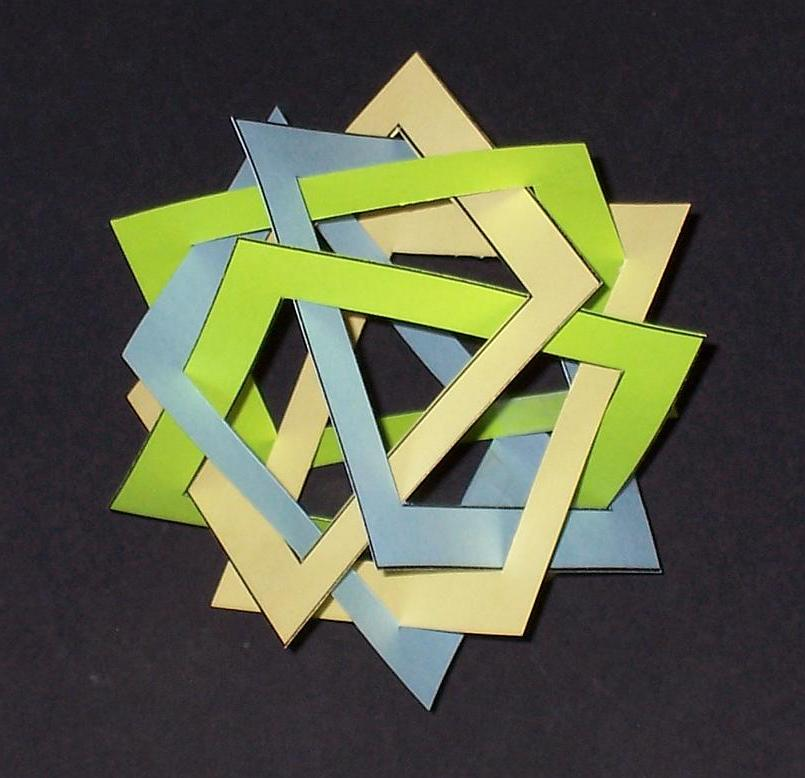Figure 1. Rotation and translation of cube faces. Figure 2. Regular polylink with six squares. Figure 3. Paper model of six linked squares.

It is instructive to create a paper model of Figure 2 by cutting out six hollow squares and interweaving them. Four of the squares can be cut open, linked through the other two, and then taped together. Figure 3 is a photo of a model made using three colors of card stock, iso-coloring parallel faces. It is chiral so one must choose between two enantiomorphs. The only critical parameter is the ratio of the edge length of the outer square to the inner square hole. By means of the software described below, a ratio of approximately 15/11 is determined to be suitable. So the outer square can be 3.7 inches on edge with a 2.7-inch square hole, leaving 0.5 inch of solidity on four sides, and four fit within an 8.5 by 11 inch sheet. This is easily drafted and cut out from paper. Or simply make six enlarged photocopies of Figure 4. For even larger models, use cardboard. Figure 5 has the same weaving as Figure 3, but the strut cross section is made into squares, to give it enough internal substance to hold together when fabricated as a solid freeform fabrication model. This fused deposition model (FDM) is 2.5 inches in diameter.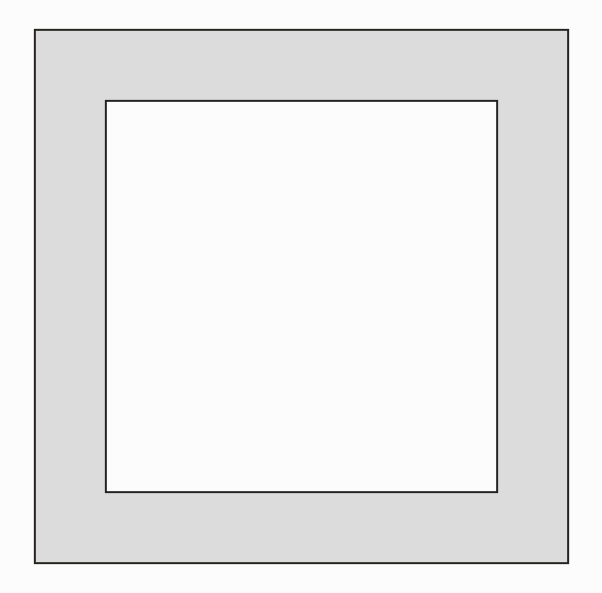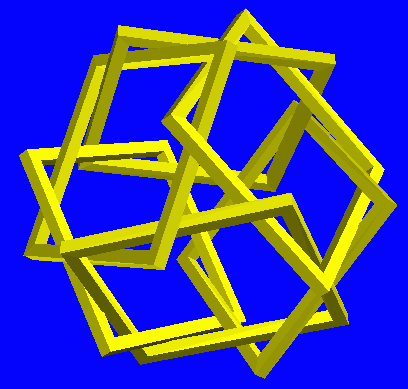Figure 4. Hollow square template for making model in Figure 3. Figure 5. FDM model with square strut cross sections. Figure 6. A different weaving of six squares, also based on cube.

Holden’s regular polylinks are the topologically distinct polygon linkages that result from varying the rotation and radial translation starting with the faces of any of the five Platonic solids. There can be several distinct ways of linking the rotated faces from any underlying polyhedron. Figure 6 shows a second way of linking six squares, but they still lie in the face planes of an imagined cube. Starting from a dodecahedron, one regular polylink of twelve pentagons is shown in Figure 7.

The struts which form the polygon edges in Figures 1-3 have rectangular cross-sections, and in Figures 5-7 they have square cross sections. Holden made his models using ¼-inch diameter wooden dowels of circular cross section. The critical dimension for a snug fit is the ratio of the strut length to diameter. He experimented until he found the shortest lengths that could be assembled, and gave a table of his results for others who replicate the constructions with round dowels . But different dimension ratios are needed if one prefers square, rectangular, or other cross sections.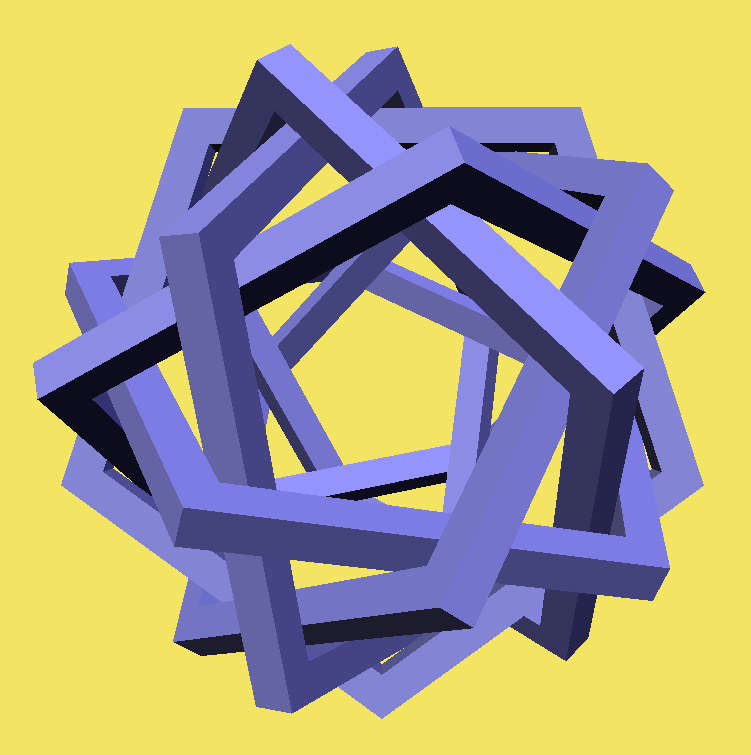Figure 7. Regular polylink with twelve pentagons. Figure 8. Regular polylink with six pentagons. Figure 9. Paper model of Fig. 8.

Figure 8 illustrates a special case to consider. The faces of a dodecahedron are translated in to a distance of zero from the center. Then by proper choice of rotation angle, it is possible to cause opposite faces of the polyhedron to coincide. So the twelve faces of the dodecahedron fuse into six concentric interwoven pentagons of figure 8. A paper model of this, Figure 9, is made from pentagons of 3-inch edge, having pentagonal holes of 2.5-inch edge. Analogously, the six faces of a cube can be translated to the origin where they fuse into three orthogonal concentric squares. But because squares have an even number of sides, they intersect other squares (in either of two rotations) rather than forming a weave. With pentagons or triangles a non-self-intersecting polylink can be formed.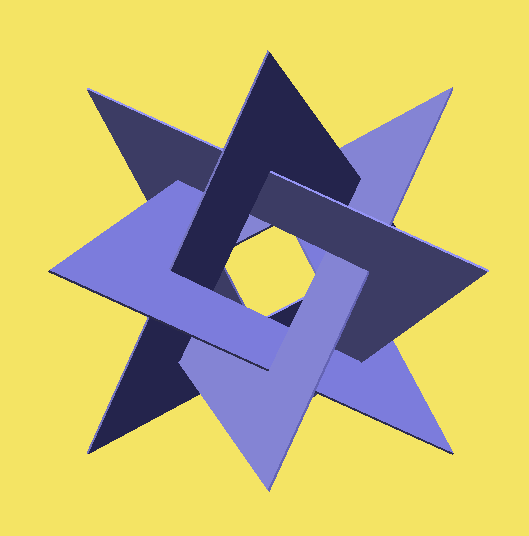Figure 10. Regular polylink composed of four triangles. Figure 11. Paper 5-inch triangles, 2.5-inch holes, seen on 3-fold axis. Figure 12. Three nested regular polylinks, each like Figure 8.

The symmetric intricacy attainable with simple components makes polylinks very appealing aesthetically. Holden illustrates only small cardboard models and dowel models, but he suggests their use in "constructivist sculpture". I have run into a dozen or so examples of sculptures based on his suggestion or a rediscovery of the essential ideas, and there are many ways to adapt, combine, or extend them. For example, Robert Lang, George Odom, Rinus Roelofs, and Carlo Sequin have explored the minimalist construction of four triangles shown in Figure 10 [1, 8, 9, 10, 11]. It can be derived from either the tetrahedron or the octahedron by translating the triangles in to the origin. Deriving Figure 10 from a tetrahedron shows that a degree of rotational freedom remains. Starting from the octahedron explains its axis of 4-fold symmetry; a particular rotation angle causes faces to merge in pairs. Coxeter analyzed this construction and showed: (1) if made of zero-thickness material, the hole in each triangle has exactly half the edge length of the whole triangle, and (2) the twelve outer vertices lie at the midpoints of a cube’s edges, i.e., the vertices of an Archimedean cuboctahedron . I recommend the reader make a paper model, as in Figure 11.

A very large polylink sculpture is Charles Perry’s 1976, twelve ton "Da Vinci", based on the six-pentagon polylink of Figure 9. See , plate D for a figure. Perry made flat steel pentagons 20-feet on a side, and nested two copies of the construction together. Figure 12 illustrates this idea but takes it further to have three concentric copies. The series can be extended inward to any depth because the components are progressively reduced in size geometrically towards the center.

Holden does not define orderly tangle precisely, but uses it loosely to subsume a variety of interesting forms such as highway interchanges, woven cloth, and polylinks. A form such as Figure 12 is not a regular polylink (because it is three regular polylinks) but it fits under the broader heading of orderly tangle.

2. Puzzles

A wooden puzzle based on the twelve pentagons of Figure 7 is shown in Figure 13. In Figure 14 is a wooden puzzle made of 30 identical sticks, which form ten triangles. It is based on an icosahedron, with the 20 faces translated to the center and rotated to coincide into ten pairs. In both puzzles, the square wooden sticks are cut longer than the polygon edges, and are notched to lock together gluelessly with half lap joints. I received these beautiful works as presents during a trip to Taiwan in July 2004. They were cleverly designed by Teacher Lin and expertly constructed by Sculptor Wu, both members of the Kaohsiung Puzzle Club. I received them disassembled for easy transport in my luggage and had to assemble them on arriving home.Figure 13. Wooden puzzle by Lin and Wu. Figure 14. Wooden puzzle by Lin and Wu.

This puzzle idea of Lin and Wu can be applied to many other polylinks if one can determine the proper length to cut the wooden sticks. As a simple example, the polylink shown in Figure 5 can be built from square stock if the ratio of the outer edge to the cross-section edge is 9.8 to 1. This value comes from the software described in the next section, but as wood is flexible and compressible, some experimenting was still required. So from 1-unit square stock, one can cut 24 pieces, each 11.8 units long. Near each end, but from opposite sides, notch halfway through to leave a 1-unit overhang beyond the notch. Figure 15 shows the assembled result. Figure 16 is the analogous puzzle of thirty sticks assembled into six pentagons woven as in Figure 8. I built both of 0.5-inch square wood bars.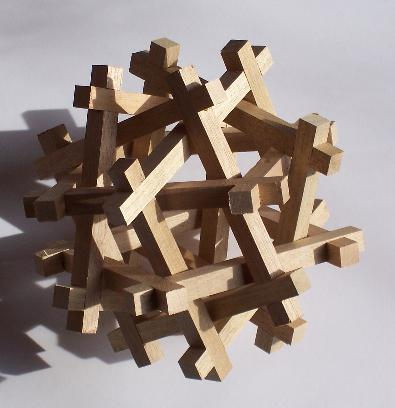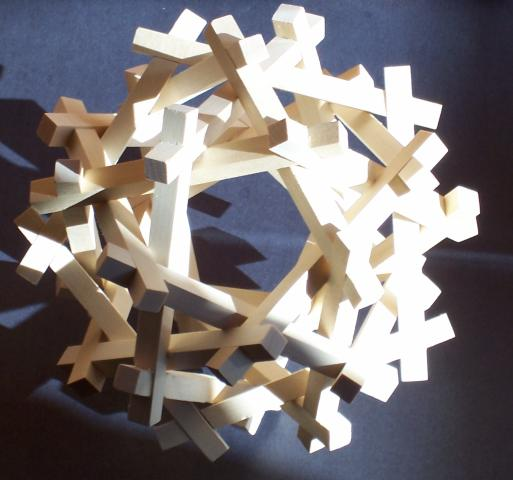Figure 15. Puzzle based on Figures 3 and 5. Figure 16. Puzzle based on Figure 8.

In these two experiments, I found that cutting the lengths a few percent shorter than the software suggests seems to compensate for the flexibility of the struts and the fact that the corners of wood struts may be compressed. So although the software provides a good initial value, the woodworker is advised to plan on some experimentation with scrap wood before investing in quality wood.

3. Software

To design and build regular polylinks and tangles of concentric polylinks, I wrote a program with sliders that can be adjusted to see a wide range of structures on-screen. The computer-generated images in this paper are from screen-shots of its operation. The user can specify any number of polylinks to be assembled concentrically, and for each set, the user selects the underlying polyhedron used as its basis. Then sliders allow the size, translation, and rotation of the components to be adjusted. At present, the edge cross sections are adjustable rectangles, allowing flat (paper) versions as the special case of width zero. As the sliders are adjusted, the dimensions of length, width, thickness, etc. are displayed, which can be used for making models of wood or other materials. When the user is happy with the form shown rotating on the screen, a click of a button generates an STL file for making solid freeform fabrication models, e.g., Figure 5. Clicking another button generates an image file that can be printed for making paper or cardboard models, e.g., Figure 4.

Three 4-cm examples made by selective laser sintering (SLS) from the program’s STL output are shown below. Figures 17 is ten triangles, arranged as in Figure 14, but with a "tall" cross-section. Figure 18 is twenty icosahedrally arranged triangles, with the minimum possible linkage (analogous to the cubic form in Figure 6). Figure 19 is a weave based on the octahedron but with the triangles replaced by hexagons.Figure 17. 10 triangle SLS model, based on icosahedron. Figure 18. 20 triangle SLS model, based on icosahedron. Figure 19. 8 hexagon SLS model, based on octahedron.

The software is freely available online at my website . It has only been tested on PCs, but it is written in java so should be transportable to other computing environments. For rendering 3D images on the screen, it uses Sun’s freely available java3D extension, which must be installed on the user’s computer. The coding is quite straightforward, with no clever algorithms or data structures needed. I believe it can be straightforwardly replicated by any software engineer with expertise in graphics programming.

Users may verify their understanding of its capabilities by replicating the figures of this paper, or one can simply start playing to create new objects. The software generates all regular polylinks including several icosahedral examples that Holden did not describe. Lang enumerated the regular polylinks with a computer search and showed there are 2 tetrahedral, 2 cubic, 3 octahedral, 5 dodecahedral, and 23 icosa-hedral varieties (excluding the extreme cases where polygons either are not linked or are merged into pairs) . I can replicate these numbers with an independent geometric technique of counting subsets of symmetry axes that pierce the interior of a polygon centrally placed in the stellation diagram.

Many extensions of the program are possible. It could be straightforwardly modified to produce circular, triangular, or other shapes of cross sections for the polygon edges. Another possible addition to the software is a numerical search for slider settings that result in a snug fit. I planned for this feature when originally designing the software, but then discovered that it is simple to see on the screen if there are gaps or overlaps in the components, so manual adjustment seems sufficient.

4. Variations

There are an unlimited number of variations on the above ideas. For example, Holden made several models of linked polygonal stars instead of convex polygons. Similarly, one might try rectangles or rhombs instead of regular polygons; I have not implemented any of these with software. (For related rectangle linkages, see .) Another idea is to start with polyhedra other than the Platonic solids. Holden constructed examples based on Archimedean solids, e.g., the rhombicuboctahedron and the snub cube. One can symmetrically combine multiple polylinks based on different polyhedra; sets with the same symmetry combine to form a tangle with the same symmetry. Figure 20 shows the combination of a cubical and a tetrahedral form. It combines the tangles of Figure 2 and Figure 10 so each triangle links three squares while each square links two triangles. Figure 21 shows the union of a dodecahedral and an icosahedral form in which both puzzles of Figures 13 and 14 are intertwined.Figure 20. 6 squares plus 4 triangles. Figure 21. 12 pentagons plus 10 triangles.

Another variation Holden tried was to put squares around the twelve 2-fold axes of a cube, i.e., in the planes of a rhombic dodecahedron. Here the squares only exhibit 2-fold symmetry, so their edges and vertices are not all equivalent, and the polylink is not regular. I find the results less attractive—fun to generate with the software but less interesting to look at. An example is shown in Figure 22. Here the twelve squares are grouped together as the sides of four triangular prisms. Extending his idea, it is natural to try putting thirty squares around the 2-fold axes of an icosahedron, i.e., in the planes of a rhombic triacontahedron. These can be grouped in many interesting ways, including five cubes or six pentagonal prisms. In Figure 23, the thirty squares are grouped together as the sides of ten triangular prisms. (When brought to the origin and fused into fifteen squares, they form five octahedra.)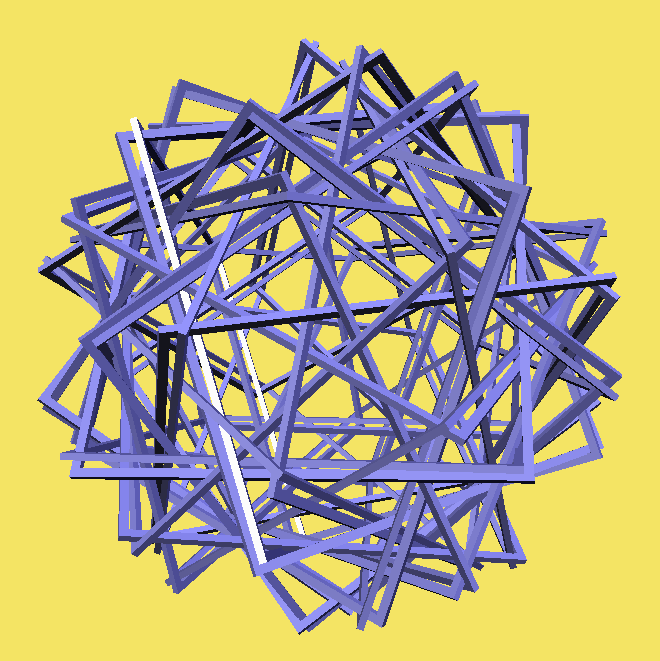Figure 22. 12 squares, in four triangular prisms. Figure 23. 30 squares in ten triangular prisms.

Spiraling the faces in a series of concentric arrangements is another idea that can be explored with the software. Fig 24 shows a simple example based on an inner cube that is surrounded by nine layers, each slightly larger and rotated five degrees more than the previous. At the end of nine steps, the 45 degree rotation of the squares leads them to be arranged as in a cuboctahedron. This is not technically a "tangle" as no polygons are linked, but it is easy to generate other examples that are. Figure 25 shows an assemblage of triangles that smoothly rotate between an outer stella octangula and an inner form of four triangles, positioned as in Figure 4. Figure 26 shows a linked set of ten triangular helixes that each join a pair of opposite faces of the outer icosahedron. Charles Perry has explored this "face screwing" concept to great effect in a number of monumental sculptures, e.g. his 1973, 35-foot tall, "Eclipse", which starts with an inner dodecahedron. See , plate D for a figure.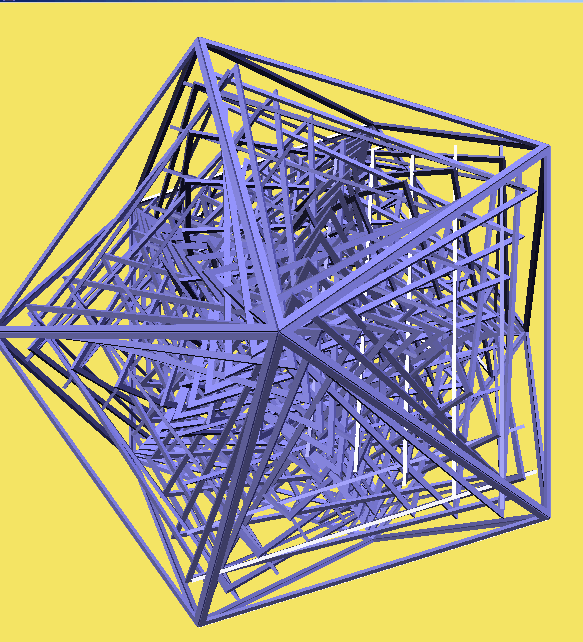Figure 24. Ten sets of squares: inner cube, outer cuboctahedron. Figure 25. Set of triangles join opposite faces of stella octangula Figure 26. Sets of triangles join opposite faces of icosahedron.

Weaving is another way to generate variations on a polylink. The oldest example that I know of a form closely related to a regular polylink is the sepaktakraw ball, seen in Figure 27. Traditionally woven from rattan, it exhibits the dodecahedral pattern of figure 8. The design goes back centuries for use in traditional Asian "football" games. Figure 28 shows a "spherical basket" I wove of paper strips. The six dark central bands follow the same weave pattern of six pentagons shown in Figure 8. Neighboring bands, which get progressively lighter in color, simply alternate over and under in the natural weave pattern. Note the difference between this weave and the sepaktakraw ball. In Figure 28 the individual strands weave, while in Figure 27 the group of strands weaves as a whole.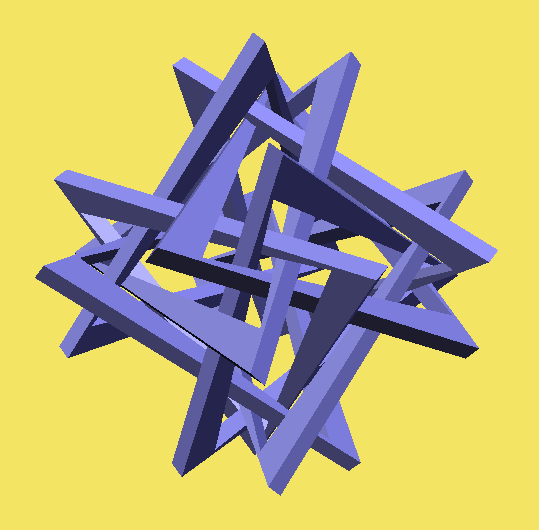Figure 27. Sepaktakraw ball Figure 28. Paper weave. Figure 29. Skew holes.

Another variation, illustrated in Figure 29, is to allow a parameter for a relative rotation between the polygon and its hole. This adds a dynamic visual quality to the forms.

A different type of variation is to replace the straight edges of the polygons with curved paths. There are infinitely many ways to choose curves, but a particularly natural one is to perform an inversion about the center of symmetry. Central inversion replaces each point at distance r from the origin with a point in the same direction but at distance 1/r. This transformation is well studied mathematically but little used in sculpture . In this context, the chain of n rectangular struts that form the edges of an n-gon is replaced by a chain of n curved volumes bounded by four portions of spheres. Figure 30 shows an example in which a square-cross-section version of the four-triangle construction in Figure 10 is inverted into a structure that resembles four interlocked three-leaf clovers. The twelve corners are now on the inside, but remain 60-degree angles because central inversion is an angle-preserving transformation.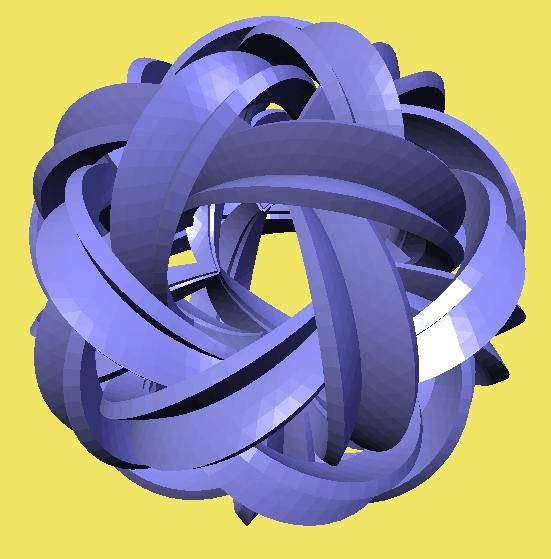Figure 30. Inversion of four triangles. Figure 31. Five tetrahedra, an icosahedral polylink. Figure 32. Inversion of five tetrahedra.

Figure 31 is the well-known compound of five regular tetrahedra, which is easily generated as an icosahedral polylink. It is formed here with struts of rectangular cross section that overlap to make ribbed edges. These invert into the labial forms of Figure 32. The interior regions of Figures 30 and 32 are very interesting spaces, difficult to capture in a still image.

5. Conclusion

Regular polylinks are a rich source of fundamental forms that may be used as the basis for a gamut of 3D design ideas. Alan Holden’s 1983 book abounds with creative inspirations displaying their symmetric elegance. But the spectrum of examples presented there and expanded upon here only scratch the surface. To introduce polylinks in a concrete manner, paper constructions such as Figures 3, 9, and 11 make a good hands-on activity. After that, I hope that the polylink-generation software described here will enable readers to explore new possibilities.

References

 H. Burgiel, D.S. Franzblau, and K.R. Gutschera, "The Mystery of the Linked Triangles," Mathematics Magazine, v. 69 (1996) 94-102.

 H.S.M. Coxeter, "Symmetric Combinations of Three or Four Hollow Triangles," Math. Intel. 16 (1994) 25-30.

 Michele Emmer (editor), The Visual Mind, MIT, 1993.

 George W. Hart, http://www.georgehart.com

 Alan Holden, Shapes, Spaces and Symmetry, Columbia Univ. Pr, 1971, (Dover reprint, 1991).

 Alan Holden, "Regular Polylinks," Structural Topology, No. 4, 1980, pp. 41-45.

 Alan Holden, Orderly Tangles: Cloverleafs, Gordian Knots, and Regular Polylinks, Columbia U., 1983.

 Robert J. Lang, "Polypolyhedra in Origami" in Thomas Hull (ed.) Origami 3, A.K. Peters, 2002.

 Rinus Roelofs, http://www.rinusroelofs.nl

 Doris Schattschneider, "Coxeter and the Artists: two-way inspiration", in The Coxeter Legacy—Reflections and Projections, (ed. C. Davis and E.W. Ellers), Fields Inst. Comm. v.46, Amer. Math. Soc., 2005. (to appear)

 Carlo Sequin, "Analogies from 2D to 3D, Exercises in Disciplined Creativity," Proc. of Bridges: Mathematical Connections in Art, Music, and Science, Winfield KS, Aug, 1999, and in Visual Math., v. 3, no. 1.

 John Sharp, "Two Perspectives on Inversion", Meeting Alhambra, Barrallo et al. (ed), 2003.

Acknowledgments: Thank you RJT Educational Training (www.rjtedu.com) for making the model of Figure 5, and thank you Jim Quinn for making the models of Figures 17-19.